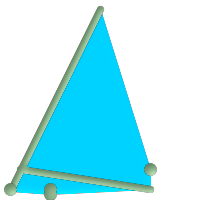## Name

ST_ConvexHull — Computes the convex hull of a geometry.

## Synopsis

`geometry ST_ConvexHull(`geometry geomA`)`;

## Description

Computes the convex hull of a geometry. The convex hull is the smallest convex geometry that encloses all geometries in the input.

In the general case the convex hull is a Polygon. The convex hull of two or more collinear points is a two-point LineString. The convex hull of one or more identical points is a Point.

It is usually used with Multi* and GeometryCollections. It is not an aggregate function. To compute the convex hull of a set of geometries, use ST_Collect to aggregate them.

One can think of the convex hull as the geometry obtained by wrapping an elastic band around a set of geometries. This is different from a concave hull which is analogous to "shrink-wrapping" the geometries. A convex hull is often used to determine an affected area based on a set of point observations.

Performed by the GEOS moduleThis method implements the OpenGIS Simple Features Implementation Specification for SQL 1.1. s2.1.1.3This method implements the SQL/MM specification. SQL-MM 3: 5.1.16This function supports 3d and will not drop the z-index.

## ExamplesConvex Hull of a MultiLinestring and a MultiPoint

```SELECT ST_AsText(ST_ConvexHull(
ST_Collect(
ST_GeomFromText('MULTILINESTRING((100 190,10 8),(150 10, 20 30))'),
ST_GeomFromText('MULTIPOINT(50 5, 150 30, 50 10, 10 10)')
)) );
---st_astext--
POLYGON((50 5,10 8,10 10,100 190,150 30,150 10,50 5))
```

Using with ST_Collect to compute the convex hulls of geometry sets.

```--Get estimate of infected area based on point observations
SELECT d.disease_type,
ST_ConvexHull(ST_Collect(d.the_geom)) As the_geom
FROM disease_obs As d
GROUP BY d.disease_type;
```